Courses

# Introduction To Belt Drives Mechanical Engineering Notes | EduRev

## Mechanical Engineering : Introduction To Belt Drives Mechanical Engineering Notes | EduRev

The document Introduction To Belt Drives Mechanical Engineering Notes | EduRev is a part of the Mechanical Engineering Course Machine Design.
All you need of Mechanical Engineering at this link: Mechanical Engineering

Flexible Machine Elements
Belt drives are called flexible machine elements. Flexible machine elements are used for a large number of industrial applications, some of them are as follows.
1. Used in conveying systems Transportation of coal, mineral ores etc. over a long distance
2. Used for transmission of power. Mainly used for running of various industrial appliances using prime movers like electric motors, I.C. Engine etc.
3. Replacement of rigid type power transmission system. A gear drive may be replaced by a belt transmission system

Flexible machine elements has got an inherent advantage that, it can absorb a good amount of shock and vibration. It can take care of some degree of misalignment between the driven and the driver machines and long distance power transmission, in comparison to other transmission systems, is possible. For all the above reasons flexible machine elements are widely used in industrial application. Although we have some other flexible drives like rope drive, roller chain drives etc. we will only discuss about belt drives.

Typical belt drives
Two types of belt drives, an open belt drive, (Fig. 13.1.1) and a crossed belt drive (Fig. 13.1.2) are shown. In both the drives, a belt is wrapped around the pulleys. Let us consider the smaller pulley to be the driving pulley. This pulley will transmit motion to the belt and the motion of the belt in turn will give a rotation to the larger driven pulley. In open belt drive system the rotation of both the pulleys is in the same direction, whereas, for crossed belt drive system, opposite direction of rotation is observed.

Nomenclature of Open Belt Drive

dL - Diameter of the larger pulley
dS – Diameter of the smaller pulley
αL- Angle of wrap of the larger pulley
αS – Angle of wrap of the smaller pulley
C- Center distance between the two pulleys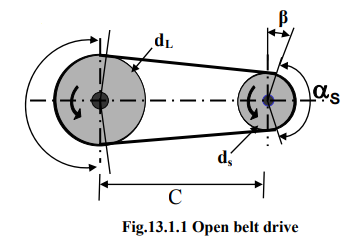Basic Formulae
αL = 180ο + 2β
αS = 180ο - 2β

Where angle β is,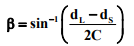L0 = Length of open belt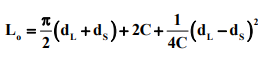This formulae may be verified by simple geometry.

13.1.4 Nomenclature of Cross Belt Drive
dL - Diameter of the larger pulley
dS – Diameter of the smaller pulley
αL- Angle of wrap of the larger pulley
αS – Angle of wrap of the smaller pulley
C- Center distance between the two pulleys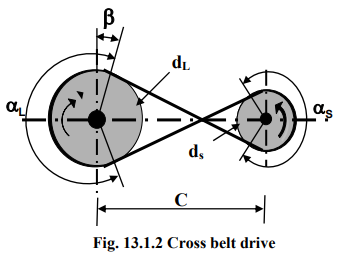Basic Formulae
α= αS = 180ο + 2β

Where angle β is,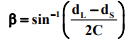Length of cross belt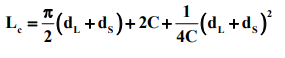Belt tensions

The belt drives primarily operate on the friction principle. i.e. the friction between the belt and the pulley is responsible for transmitting power from one pulley to the other. In other words the driving pulley will give a motion to the belt and the motion of the belt will be transmitted to the driven pulley. Due to the presence of friction between the pulley and the belt surfaces, tensions on both the sides of the belt are not equal. So it is important that one has to identify the higher tension side and the lower tension side, which is shown in Fig. 13.1.3.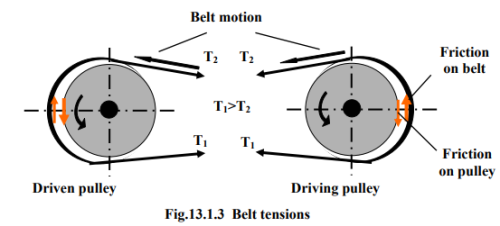When the driving pulley rotates (in this case, anti-clock wise), from the fundamental concept of friction, we know that the belt will oppose the motion of the pulley. Thereby, the friction, f on the belt will be opposite to the motion of the pulley. Friction in the belt acts in the direction, as shown in Fig. 13.1.3, and will impart a motion on the belt in the same direction. The friction f acts in the same direction as T2. Equilibrium of the belt segment suggests that T1 is higher than T2. Here, we will refer T1 as the tight side and T2 as the slack side, ie, T1 is higher tension side and T2 is lower tension side.

Continuing the discussion on belt tension, the figures though they are continuous, are represented as two figures for the purpose of explanation. The driven pulley in the initial stages is not rotating. The basic nature of friction again suggests that the driven pulley opposes the motion of the belt. The directions of friction on the belt and the driven pulley are shown the figure. The frictional force on the driven pulley will create a motion in the direction shown in the figure. Equilibrium of the belt segment for driven pulley again suggests that T1 is higher than T2.

It is observed that the slack side of the belt is in the upper side and the tight side of the belt is in the lower side. The slack side of the belt, due to self weight, will not be in a straight line but will sag and the angle of contact will increase. However, the tight side will not sag to that extent. Hence, the net effect will be an increase of the angle of contact or angle of wrap. It will be shown later that due to the increase in angle of contact, the power transmission capacity of the drive system will increase. On the other hand, if it is other way round, that is, if the slack side is on the lower side and the tight side is on the upper side, for the same reason as above, the angle of wrap will decrease and the power transmission capacity will also decrease. Hence, in case of horizontal drive system the tight side is on the lower side and the slack side is always on the upper side.

Derivation of relationship between belt tensions

The Fig.13.1.4 shows the free body diagram of a belt segment.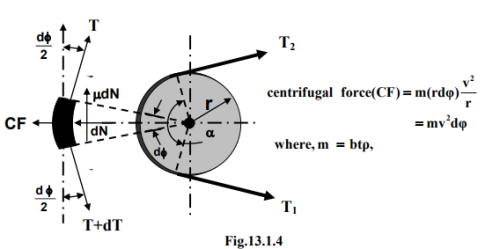The belt segment subtends an angle dφ at the center. Hence, the length of the belt segment,

dl = r dφ                         (13.1.1)

At the impending condition, ie., when the belt is in just in motion with respect to the pulley, the forces acting on the belt segment are shown in Fig.13.1.4. This belt segment is subjected to a normal force acting from the pulley on the belt segment and due to the impending motion the frictional force will be acting in the direction as shown in the figure

. f = μdl                        (13.1.2)

where μ is the coefficient of friction between the belt and the pulley. The centrifugal force due to the motion of the belt acting on the belt segment is denoted as CF and its magnitude is,

CF = [m(rdφ)x v2 ]/r = mv2 dφ             (13.1.3)

Where, v is the peripheral velocity of the pulley m is the mass of the belt of unit length,

m = btρ                         (13.1.4)

where, b is the width, t is the thickness and ρ is the density of the belt material. From the equation of equilibrium in the tangential and normal direction,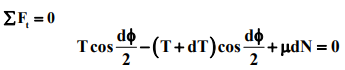(13.1.5)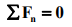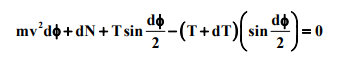(13.1.6)

For small angle, dφ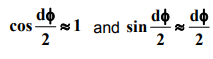Therefore, simplified form of (13.1.5) is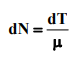(13.1.7)

From (13.1.6) and using (13.1.7),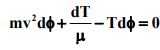or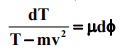(13.1.8)

Considering entire angle of wrap,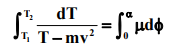(13.1.9)

The final equation for determination of relationship between belt tensions is,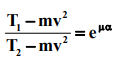(13.1.10)

It is important to realize that the pulley, driven or driver, for which the product, μα of (13.1.10) is the least, should be considered to determine the tension ratio. Here, α should be expressed in radians.

Elastic Creep and Initial Tension

Presence of friction between pulley and belt causes differential tension in the belt. This differential tension causes the belt to elongate or contract and create a relative motion between the belt and the pulley surface. This relative motion between the belt and the pulley surface is created due to the phenomena known as elastic creep.

The belt always has an initial tension when installed over the pulleys. This initial tension is same throughout the belt length when there is no motion. During rotation of the drive, tight side tension is higher than the initial tension and slackside tension is lower than the initial tension. When the belt enters the driving pulley it is elongated and while it leaves the pulley it contracts. Hence, the driving pulley receives a larger length of belt than it delivers. The average belt velocity on the driving pulley is slightly lower than the speed of the pulley surface. On the other hand, driven pulley receives a shorter belt length than it delivers. The average belt velocity on the driven pulley is slightly higher than the speed of the pulley surface.

Let us determine the magnitude of the initial tension in the belt.

Tight side elongation ∝ (T1 – Ti
Slack side contraction ∝ (Ti – T2 )

Where, Ti is the initial belt tension .

Since, belt length remains the same, ie, the elongation is same as the contraction,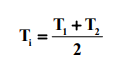(13.1.11)

It is to be noted that with the increase in initial tension power transmission can be increased. If initial tension is gradually increased then T1 will also increase and at the same time T2 will decrease. Thus, if it happens that T2 is equal to zero, then T1 = 2Ti and one can achieve maximum power transmission.

Velocity ratio of belt drive

Velocity ratio of belt drive is defined as,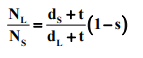where,

NL and NS are the rotational speeds of the large and the small pulley respectively, s is the belt slip and t is the belt thickness.

Power transmission of belt drive

Power transmission of a belt drive is expressed as,

P = ( T1 – T2 )v                   (13.1.13)

where, P is the power transmission in Watt and v is the belt velocity in m/s.

Sample problem A pump is driven by an electric motor through a open type flat belt drive. Determine the belt specifications for the following data.

Motor pulley diameter(dS) = 300 mm, Pump pulley diameter(dL) = 600 mm
Coefficient of friction (μS) for motor pulley = 0.25
Coefficient of friction (μL) for pump pulley = 0.20
Center distance between the pulleys=1000 mm; Rotational speed of the motor=1440 rpm;
Power transmission = 20kW; density of belt material (ρ)= 1000 kg/m3 ; allowable
| stress for the belt material (σ) = 2
MPa; thickness of the belt = 5mm.

Solution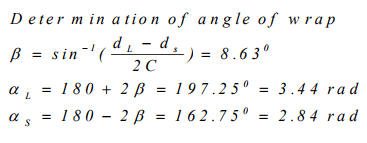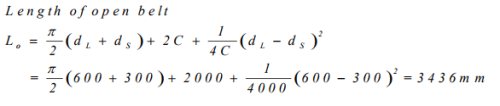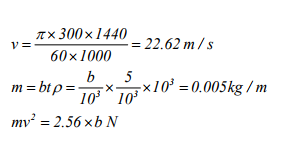.............................. (1)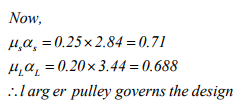......................................... (2)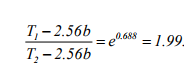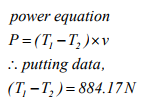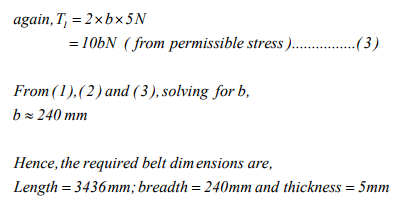Offer running on EduRev: Apply code STAYHOME200 to get INR 200 off on our premium plan EduRev Infinity!

## Machine Design

57 videos|71 docs|102 tests

,

,

,

,

,

,

,

,

,

,

,

,

,

,

,

,

,

,

,

,

,

;